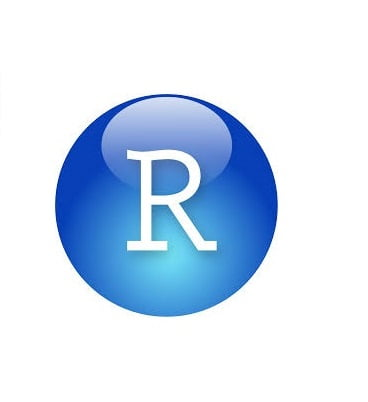14 - 02 - 2019

## Happy Valentines day by Nerds!

Real nerds on Valentines day graph hearts instead of drawing them. My drawing skills are not what I like them to be, my R skills are though! Therefore, let’s draw a heart in R instead on paper!
```dat<- data.frame(t=seq(0, 2*pi, by=0.01) )
xhrt<- function(t) 16*sin(t)^3
yhrt<- function(t) 13*cos(t)-5*cos(2*t)-2*cos(3*t)-cos(4*t)
dat\$y=yhrt(dat\$t)
dat\$x=xhrt(dat\$t)
```
```with(dat, plot(x,y, type="l", axes=FALSE, frame.plot=FALSE, labels = FALSE, xlab = '', ylab = ''))
with(dat, polygon(x,y, col="#FF7575"))
```

Inspired by: http://mathworld.wolfram.com/HeartCurve.html